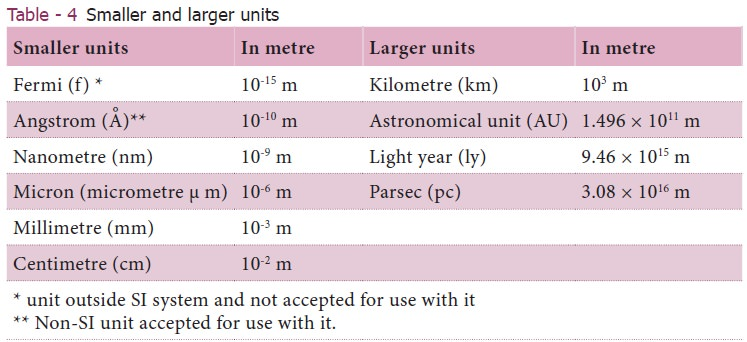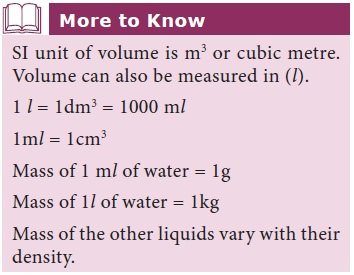Home | | Science 9th std | Fundamental Units of SI System

# Fundamental Units of SI System

Length is de ned as the distance between two points. The SI unit of length is metre.

Fundamental Units of SI System

## 1. Length

Length is de ned as the distance between two points. The SI unit of length is metre. One metre is the distance travelled by light through vacuum in 1/29,97,92,458 second.

In order to measure very large distance (distance of astronomical objects) we use the following units.·        Light year

·        Astronomical unit

·        Parsec

Light year: It is the distance travelled by light in one year in vacuum and it is equal to 9.46 × 1015m.

Astronomical unit (AU): It is the mean distance of the centre of the Sun from the centre of the earth. 1 AU = 1.496 × 1011 m Figure 1.Parsec: Parsec is the unit of distance used to measure astronomical objects outside the solar system.

1 parsec = 3.26 light year.

To measure small distances such as distance between two atoms in a molecule, the size of the nucleus and the wavelength, we use submultiples of ten. These quantities are measured in Angstrom unit (Table 4).## 2. Mass

Mass is the quantity of matter contained in a body. The SI unit of mass is kilogram. One kilogram is the mass of a particular international prototype cylinder made of platinum-iridium alloy, kept at the International Bureau of Weights and Measures at Sevres, France.The related units in submultiples of 10 (1/10) are gram and milligram and in multiples of 10 are quintal and metric tonne.

1 quintal = 100 kg

1 metric tonne = 1000 kg = 10 quintal

1 solar mass = 2 × 1030 kg

Atomic mass unit (amu):

Mass of a proton, neutron and electron can be determined using atomic mass unit.

1 amu = 1/12th of the mass of carbon-12 atom.## 3. Time

Time is a measure of duration of events and the intervals between them. The SI unit of time is second. One second is the time required for the light to propagate 29,97,92,458 metres through vacuum. It is also defined as 1/86, 400th part of a mean solar day. Larger unit for measuring time is millennium. 1 millenium = 3.16 × 109 s.

## 4. Temperature

Temperature is the measure of hotness. SI unit of temperature is kelvin(K). One kelvin is the fraction of 1/273.16 of the thermodynamic temperature of the triple point of water ( e temperature at which saturated water vapour, pure water and melting ice are in equilibrium). Zero kelvin (0 K) is commonly known as absolute zero. The other units for measuring temperature are degree Celsius and Fahrenheit (Table 5). To convert temperature from one scale to another we use

C/100 = (F – 32)/180 = (K-273) /100

### Example:

Convert (a) 300 K in to Celsius scale, (b) 104°F in to Celsius scale.

Solution

(a) Celsius = K-273 = 300-273 = 27°C

(b) Celsius = (F – 32) × 5/9 = (104-32) × 5/9 =72 × 5/9 = 40°CStudy Material, Lecturing Notes, Assignment, Reference, Wiki description explanation, brief detail
9th Science : Measurement and Measuring Instruments : Fundamental Units of SI System |